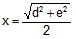Problem 399: Right triangle, Midpoints, Distance, Pythagorean theorem, Congruence

 The figure shows a right triangle ABC. F is the midpoint of DE and G is the midpoint of AC. If AD = d, CE = e, and FG = x, prove that. .Home | Search | Geometry | Problems | All Problems | 391-400 | Email | View or post a solution